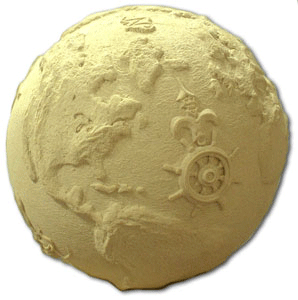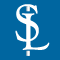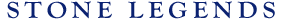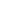HOMEPRODUCTSIDEA CENTERSERVICESTECHNICALABOUT USHello Guest Login  |  Become Member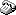800-398-1199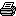214-398-1293MapEmail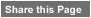Click to Share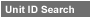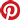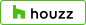Select which variables you would like to search by.Type DescriptionPrimary ViewPrimary ModifierUnit NameView Definitions

View Definitions

View DefinitionsX-Dim Y-Dim Z-Dim XX-Dim YY-Dim ZZ-Dim R-Dim D-Dim P-Dim
 Click column heading to re-sort results list.Product Name Primary View Modifier Pri Dimx  y  z Alt Dimxx  yy  zz Alt2 Dimr  d  p E\$timated Thumbnail Angelica Statuary X = 11" Y = 5"Z = 5" XX = n/a YY = n/aZZ = n/a R = n/a D = n/aP = n/a \$ 67.53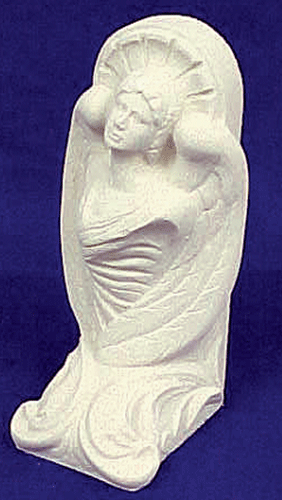Benny the Mouse Statuary X = 2" Y = 4-1/2"Z = 3-1/2" XX = n/a YY = n/aZZ = n/a R = n/a D = n/aP = n/a \$ 11.37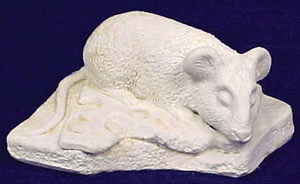Bucephalus Statuary X = 16-1/4" Y = 5-3/8"Z = 10" XX = n/a YY = n/aZZ = 7-1/4" R = n/a D = n/aP = n/a \$ 134.65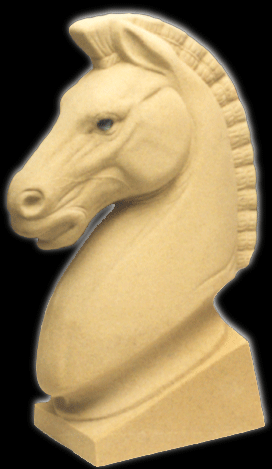Corinthian Cap Statuary X = 13" Y = 11-3/4"Z = 13" XX = n/a YY = n/aZZ = n/a R = n/a D = 8"P = n/a \$ 85.56Damon, Boy on a Dolphin Statuary X = 19" Y = 13"Z = 10-1/2" XX = n/a YY = n/aZZ = n/a R = n/a D = n/aP = n/a \$ 173.38Danae Statuary X = 20" Y = 6"Z = 2-1/2" XX = n/a YY = n/aZZ = n/a R = n/a D = n/aP = n/a \$ 55.32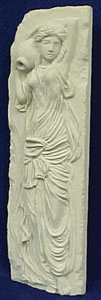Freddy the Frog 2 Statuary X = 7-1/2" Y = 7"Z = 9" XX = n/a YY = n/aZZ = n/a R = n/a D = n/aP = n/a \$ 32.58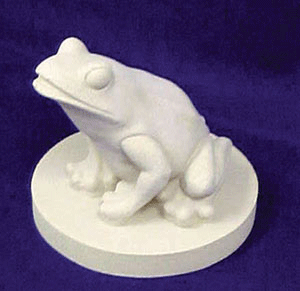Leander Statuary X = 18" Y = 21"Z = 8" XX = n/a YY = n/aZZ = n/a R = n/a D = n/aP = n/a \$ 328.23Lenore Statuary X = 13-1/4" Y = 18-3/4"Z = 8-7/8" XX = n/a YY = n/aZZ = n/a R = n/a D = n/aP = n/a \$ 412.65Lion Prone Statuary X = 33" Y = 63"Z = 27" XX = n/a YY = n/aZZ = n/a R = n/a D = n/aP = n/a \$ 1077.26Mandrake Statuary X = 7" Y = 14"Z = 6" XX = n/a YY = n/aZZ = n/a R = n/a D = n/aP = n/a \$ 135.12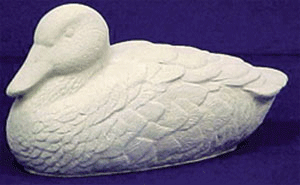Tetisheri Statuary X = 12" Y = 18"Z = 10" XX = n/a YY = n/aZZ = n/a R = n/a D = n/aP = n/a \$ 335.27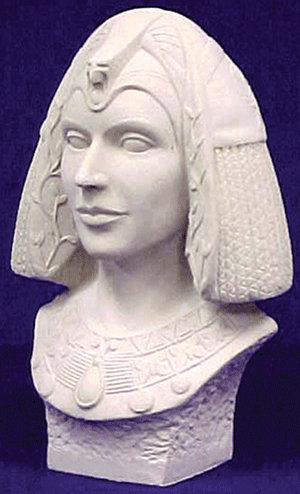Under the Sea Statuary X = 19-1/4" Y = 28"Z = 3" XX = n/a YY = n/aZZ = n/a R = n/a D = n/aP = n/a \$ 185.34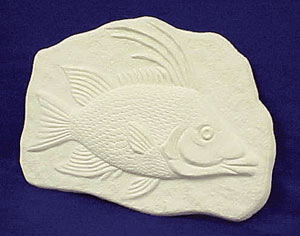Winged Lion Statuary X = 13" Y = 7"Z = 7-1/2" XX = n/a YY = n/aZZ = n/a R = n/a D = n/aP = n/a \$ 76.44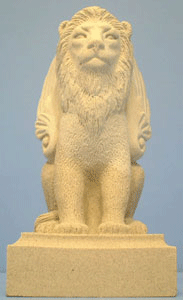World Globe Statuary X = 12" Y = 24-1/2"Z = 12" XX = n/a YY = n/aZZ = 10" R = n/a D = 12"P = n/a \$ 253.17